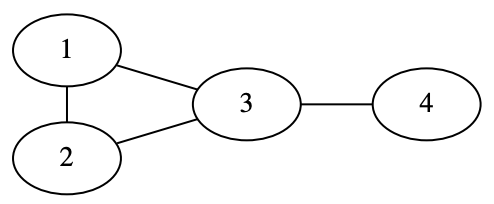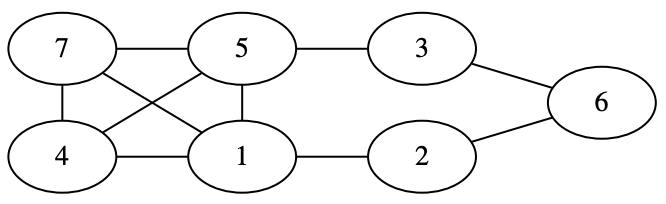시간 제한메모리 제한제출정답맞은 사람정답 비율
4 초 512 MB64121018.519%

## 문제

You are given a connected undirected unweighted graph. The distance d(u, v) between two vertices u and v is defined as the number of edges in the shortest path between them. Find the sum of d(u, v) over all unordered pairs (u, v).

## 입력

The first line of the input contains two integers n and m (2 ≤ n ≤ 105 ; n−1 ≤ m ≤ n+ 42) — the number of vertices and the number of edges in the graph respectively. The vertices are numbered from 1 to n.

Each of the following m lines contains two integers xi and yi (1 ≤ xi , yi ≤ n; xi ≠ yi) — the endpoints of the i-th edge.

There is at most one edge between every pair of vertices.

## 출력

Output a single integer — the sum of the distances between all unordered pairs of vertices in the graph.

## 예제 입력 1

4 4
1 2
2 3
3 1
3 4


## 예제 출력 1

8## 예제 입력 2

7 10
1 2
2 6
5 3
5 4
5 7
3 6
1 7
5 1
7 4
4 1


## 예제 출력 2

34## 노트

In the first example the distance between four pairs of vertices connected by an edge is equal to 1 and d(1, 4) = d(2, 4) = 2.